Editor Ratings:
User Ratings:
[Total: 0 Average: 0]

Here are 10 free websites with printable math worksheets for kindergarten that can be helpful to practice math subject. The worksheets have enough categories within this math subject that can be fun and educational. It covers categories like identifying the different shapes, tracing numbers, tally numbers, counting, identifying the time and many more activities. These math worksheets can be taken out as prints and can be provided to children for their practice.

These sites have a wide range of printable math worksheets for kindergarten. You will never run out of trying another math worksheet as they have enough variety. Children will enjoy solving math expression with these worksheets in a playful manner. With the help of these worksheets, children can take more interest in the math subject. Now kids can play and practice math simultaneously.

Let’s just go through these websites one by one.

## 1. MATH is FUN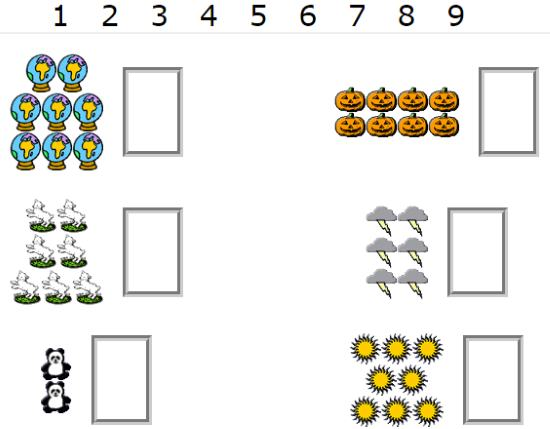MATH is FUN  is a good website that has printable math worksheets for kindergarten. The site has categories in math worksheets like counting, addition, fill in the missing numbers, etc. Children can practice these worksheets in a playful manner.

You can see in the above picture, this is the counting worksheet in which children have to count objects in the picture and then write the total number in the box next to the pictures. Children will love learning numbers by counting these objects in the worksheet.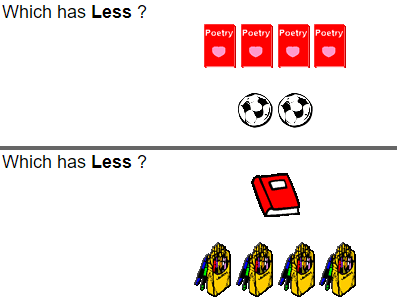There is another worksheet you can see in the above image, the worksheet has two groups of objects in each column. The children have to count which group has less objects than the other. Thereafter, children need to circle the group that has fewer objects than the other group’s items. For example, in the first column, there are 4 poetry books and 2 footballs. Here, children will circle footballs as the football group is lesser than poetry book group.

The site offers other printable math worksheets for kindergarten that can be interesting to practice. You can take out prints of these worksheets in B/W and color as per your preference.

## 2. Great! SCHOOLS.orgThe website (Great! SCHOOLS.org) has more than 100 printable math worksheets for kindergarten. Children can learn numbers by coloring the objects, adding numbers, knowing the different shapes, etc.

The above-posted picture has groups of items and each group has 2 extra items than the other group. Here, children have to identify which row has two more items then they have to check the box next to it. For example, the first row has 5 hats whereas the second row has 7 hats. Hence, children need to mark a tick in the box right after 7 hats.

There is another counting game below this where children need to count items. Thereafter, children have to add 2 more items and then write the total number in the box. For example, there are 4 hats and if we add 2 more hats with 4, the total number will become 6. Hence, children need to write number 6 in the box.

In this way, children can learn to count and add numbers in a playful manner. You can try these math worksheets for kids and get the print outs from the site itself.

## 3. KidZone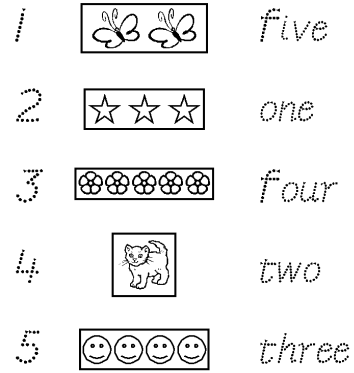KidZone is a good website that offers variable printable math worksheets for kindergarten. The site also has math games which can be interesting for kids to play.

The site has math worksheets like tracing numbers, count and color, connect the dots of counting to form a picture and so on. In the above-posted math worksheet, children have to count these items and then read the word. Thereafter, children need to match the correct word. For example, 2 butterflies will be matched with the word two. Learning these numbers and counting items can be playful for kids.

There are other math worksheets for kindergarten provided by the KidZone site. One of the worksheets I tried where children need to join numbers from 1-9 to form a picture of a duck. Thereafter, children can color the duck picture.

The site has plenty of math worksheets which can be taken out as print and provide these sheets to children for their practice.

## 4. Worksheetfun.comWorksheetfun.com is a good website for free printable math worksheets for kindergarten. The site helps children in learning numbers, recognizing different shapes like circle, rectangle, square, etc.

In the above image, children can practice before and after numbers. The above sheet has images of airplanes. Each airplane has 3 boxes with one number in the middle box. Here, children have to write what number comes before and after the given number. The first airplane numbers are already solved in the above screenshot. The second airplane has the number 5 given. The children have to write the number before which is 4 and the number after which is 6 so it becomes 4-5-6.

There are other worksheets provided by Worksheetfun.com. One of the other worksheets I tried asked to recognize the different shapes like circle, square, rectangle and triangle. Then children need to color them with different colors. In this way, children can identify various shapes along with their names.

## 5. K5 LearningK5 Learning is a simple website that provides printable math worksheets for kindergarten. The site offers worksheets like addition, subtraction, recognizing the pattern.

In the above image, you can see the math worksheet that asks kids to recognize the shapes and complete the pattern. For example, the first column has 1 triangle shape and then an oval shape. Here, children need to follow this pattern by drawing shapes and complete the worksheet.

There are other worksheets on the same site (K5 Learning). I tried another addition worksheet that asks kids to count the objects with other groups of objects and then write the total number in the box. Similarly, the subtraction works in the same way where kids need to count the objects and then subtract from the other group of objects. Thereafter, children need to write the subtraction equation in the box. In this way, children can learn the sums like addition and subtraction in an easy and playful manner.

## 6. Education.com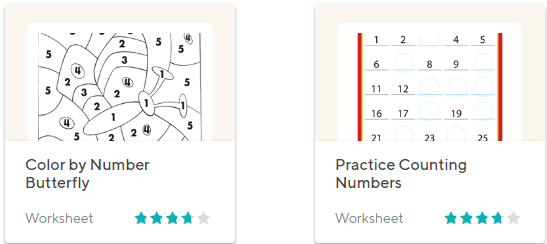Education.com is one of the famous websites that offer worksheets in variables. The site also provides printable math worksheet for kindergarten.

You can see in the above image, children can practice numbers by coloring the butterflies. Here, in this worksheet, children have to color different parts of the butterfly as it is mentioned in the worksheet. For example, all the parts of the butterfly with number 1 in them needs to filled with green color and number 2 can be filled with orange color. Similarly, all the different numbers can be filled with different colors. Ultimately, children will color the butterfly beautifully.

The other worksheet in the second picture is to practice counting numbers. Here, the worksheet has missing numbers and children need to fill an empty place with the correct number in order from 1-30.

You can take out prints of these math worksheets for kindergarten and provide to children to make their math practice easy.

## 7. Math4Children

Math4Children offers not just math worksheets but also the games through which children can practice math lessons playfully. The site has math worksheets like addition, subtraction, comparison, counting tally marks, patterns, etc.The worksheet like counting tally marks is good for kids as you can see in the above image. Here, kids need to tally marks and then write the correct number in the answer. There are other worksheets from where children can practice numbers by tracing the numbers on the worksheet.

The comparison math worksheet provides two objects of different sizes and the children need to identify which one is bigger or smaller and then children need to mark the object as per the questions.

One of the worksheets I tried on Math4Children which asked children to count the shapes and then write the correct expression in the answer column. Printouts of these math worksheets can help children to practice math with fun.

## 8. MATH-SALAMANDERS.COM

The website (MATH-SALAMANDERS.COM) has a good collection of printable math worksheets for kindergarten. The site has worksheets like comparing the sizes of objects, tracing numbers, least numbers, etc.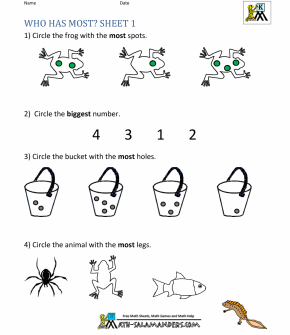In the above image, children need to solve 4 questions in this worksheet. The first question asks kids to circle the frog that has more spots on its body. The children have to count spots on each frog and then make a circle around the frog with most spots on it. In the second question, it asks children to recognize the biggest number. Here, in this picture we have numbers like 4 3 1 2 and the children have to circle the number 4 as it is the biggest number among them. The third question can simply be solved by recognizing the bucket with the most holes. Thereafter, children have to mark a circle around that bucket. In the last question, children need to mark a circle around the animal which has most legs.

There are other printable math worksheets for kindergarten available on MATH-SALAMANDERS.COM such as joining dot to dot through the numbers. Comparing the size of objects that can help children to identify different sizes of objects. You can take out prints of these math worksheets and provide to kids in order to teach them math subject playfully.

## 9. Math-Aids.Com

The website (Math-Aids.Com) has so many fun math worksheets for kindergarten. They have worksheets like shape recognition, number recognition, color the shapes, identify numbers, etc.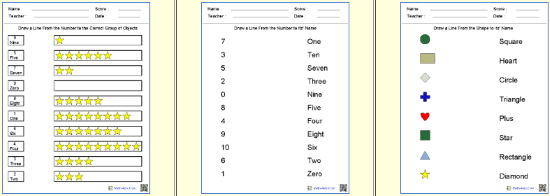You can see in the above image, the first worksheet has numbers on the left side and the other side has stars. Here, children need to match the numbers with the correct group of objects. Children will find these math worksheets fun to solve.

The second worksheet has the same method. Here, children need to simply match these numbers with their names. For example, number 7 should be matched with the word seven.

The third worksheet is to recognize different shapes and then kids need to match these shapes to their names. For example, the first shape is a circle, so children need to match this circle shape to its name Circle written on the right side in the worksheet.

You can try these printable math worksheets for kindergarten to let kids practice math in a playful way.

## 10. EDUBUZZKIDS

EDUBUZZKIDS is a good website that has multiple printable math worksheets for kindergarten. The site has quite good collection of math worksheets like addition, subtraction, comparison, time, measurement, shapes and much more.You can see in the above image, the first math worksheet asks kids to measure the size of an animal. There are good varieties within worksheets available on the site. The first worksheet has an animals like elephant and a fish, one big and one small. Here, kids have to recognize which is big or small and then they have to color the big animal from the both.

The second worksheet is about learning Time. You can see the above image, each picture has a clock with different time on it. Here, children need to recognize what time they can see in the clock and then they have to trace the time so that children can learn how to read time on a clock. This is a good way to teach children how to check time in the clock. Parents can also try these activities with kids at home.

The third worksheet has a classification of the objects. For example, a worksheet has 4 pictures of animals. Three of them are the images of ants and one is the image of an owl. Here, children need to find  the odd animal like owl and mark it.

Teachers and parent can try these printable math worksheets for kindergarten to teach math with easy methods. Kids will also practice math playfully with the help of these worksheets. Keep practicing and keep trying these worksheets and other activities. You can also read this article for math quiz websites for kids.

### In brief

These free printable math worksheets for kindergarten are best to practice. You can take out prints and provide sheets to kids. These sites can help kids in learning important things that can be knowledgeable like counting, measurement, recognizing different shapes, time and many more. Try these sites and make your children’s mind sharp and smart.

 Editor Ratings: User Ratings:[Total: 0 Average: 0] Tags: educationmath game